# Binary Search Algorithm: Fast Searching on Sorted Array!

### Let's understand the binary search problem

Given a sorted array X[] of n elements, search a given element key in X[]. If the key exists, then we need to return its index in the sorted array. Otherwise, return -1.

• All the integers in X[] are unique.
• X[] is sorted in ascending order.

Examples

Input: X[] = [-4, 2, 4, 5, 9, 12], key = 5, Output: 3

Explanation: 5 exists in X[] and its index is 3. So we return 3.

Input: X[] = [-4, 2, 4, 5, 9, 12], key = 6, Output: -1

Explanation: 6 does not exist in x[] so we return -1.

### How does the binary search algorithm works? Let's understand the binary search idea via an example!

Let's imagine a game where the computer selects a number between 1 and 16, and we need to find this number with a minimum number of guesses. The computer tells us whether the guessed number is equal to, greater, or less than the actual number for each guess.

1 2 3 4 5 6 7 8 9 10 11 12 13 14 15 16

The linear guess of all the continuous values 1, 2, . . . 16 is inefficient because, with each question, we eliminate one number only. In the worst-case scenario, we need to guess 16 times! But our aim is to find the number with a minimum number of guesses. so what would be the best guess at the start?

Here is an idea: numbers are given in sorted order, so the best first guess would be to choose the middle number (which is 8 here) and reduces the set of numbers by half in one go. Think!

• If the actual number is equal to the guessed number(8) then we are done.
• If the actual number is less than the guessed number(8) then we ignore the range of numbers from 9 to 16 in our next guess. Why? Because all the number between 9 to 16 is already greater than 8!. Now we repeat the same process for the numbers 1 to 8 and select middle number 4 as the guessed number, and so on…
• If the actual number is greater than the guessed number(8) then we ignore the range of numbers from 1 to 7 in our next guess. Why? Because all the number between 1 to 7 is already smaller than 8! Now we repeat the same process for the numbers 9 to 16 and select middle number 12 as the guessed number, and so on…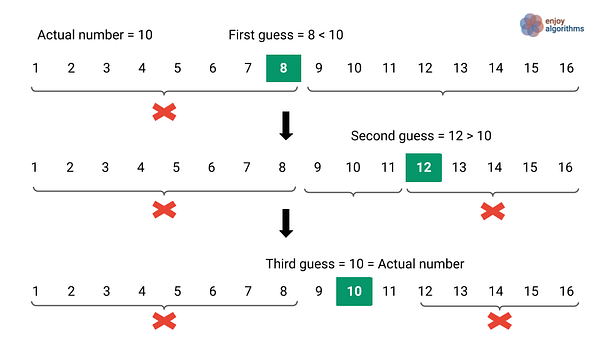After every guess, we reject half of the given numbers in one go. This is a significant improvement where we are decreasing the input size by a factor of 1/2. So what would be the minimum number of guesses required in the above problem? It's an exercise for you to think about!

### Binary search idea  of divide and conquer

The binary search algorithm is a fundamental problem to understand the divide and conquer approach: dividing a given problem into smaller sub-problems, solving each sub-problems recursively, and combining the sub-problem's solutions to obtain a solution to the original problem. But how do we apply the divide and conquer approach? Let's think!

• If the key value is less than the value at the middle index, we search the key in the left subarray, i.e., the same searching problem with input size n/2.
• Otherwise, we search the key in the right subarray, i.e., the same searching problem with input size n/2.

We recursively search until the key is found (successful search) or the subarray size is equal to 0 (unsuccessful search). The core idea is: At each stage of recursion, we have only one sub-problem and we search the key in the array by continuously dividing the search interval in half.

### Recursive algorithm of binary search

Suppose we use a function binarySearch(X[], l, r, key) to search the given value key in the sorted array. Here we are using two variables, l and r as the index of the left and right end of the subinterval in the array X[]. We start with l = 0 and r = n-1.

Divide part

• We first calculate the middle index of the array i.e., mid = l + (r - l)/2
• If (X[mid] == key), we found the value key at index mid, i.e., a case of the successful search, and we return the value mid.

Conquer part

• If the middle element is greater than the key, then the key must not be present in the right half of the array, or in other words: we recursively search the key in the left half of the array.

``````if (X[mid] > key)
return binarySearch(X, l, mid - 1, key)
``````
• If the middle element is smaller than the key, then the key must not be present in the left half of the array, or in other words: we recursively search the key in the right half of the array.

``````if (X[mid] < key)
return binarySearch(X, mid + 1, r, key)
``````
• Similarly, on the basis of comparison with the middle value, we continue searching recursively on either the left or right half of the array, again finding the middle element and proceeding as before.

Combine part

This is a trivial step because, after comparing the middle element, one sub-problem solution (either left or right) will return the index if the key is present in the array. No need to combine the solution of the sub-problems! Think!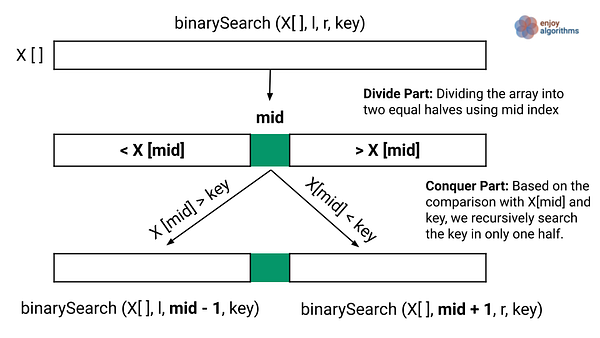Binary search base case

The base case would be the scenario when the left index crosses the right index and the subarray shrinks to zero i.e. if (l > r), return -1. This is the case of an unsuccessful search when the key is not present in the array. In other words, this would be the last stage of the recursion or the smallest version of the sub-problem!

Recursive binary search pseudocode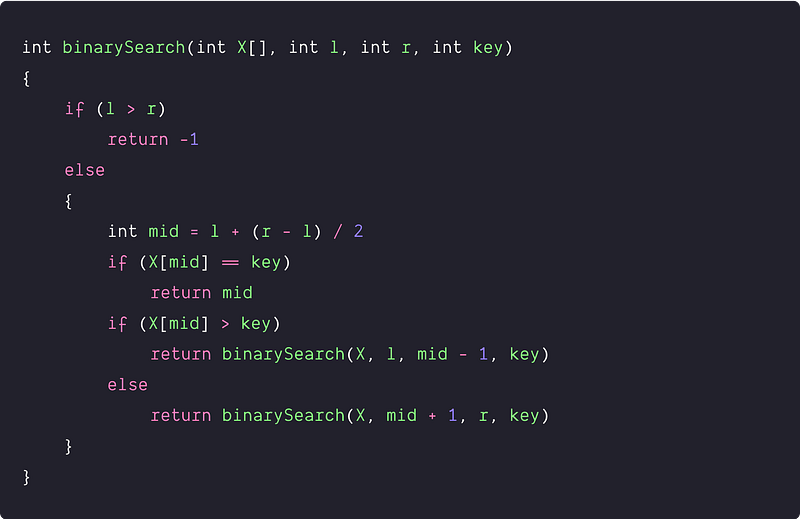### Iterative algorithm of binary search

Binary search is easy to visualize using recursion. But can we implement the above code using iteration or loop? Let’s think! If we observe closely, only two parameters get updated during every recursive call: the left and right index. In other words, we need to find a way to update the left and right index of the current sub-array in a loop. Here is the idea:

• If the middle element is greater than the key, we need to search the key in the left half of the array. For the left array, the left index would be the same, but the right index would be mid - 1.

``````if (X[mid] > key)
r = mid - 1
``````
• If the middle element is smaller than the key, we need to search the key in the right half of the array. For the right array, the right index would be the same, but the left index would be mid + 1.

``````if (X[mid] < key)
l = mid + 1
``````
• Similarly, after comparison with the mid-value, we continue searching iteratively on either the left or right half of the array, again finding the middle element and proceeding as before. Loop will stop if we either find the key (Successful search) or if the size of the subarray shrinks to zero (l > r), which is a case of unsuccessful search). In other words, the loop will continue till (l <= r).

Pseudocode of iterative binary search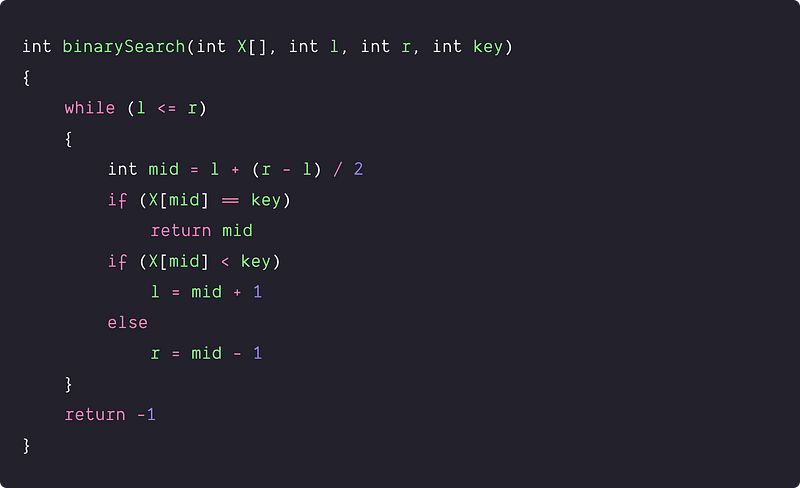### Time complexity analysis of binary search

After each comparison in the algorithm, the size of the input decreases by half. Worst case situation would be an unsuccessful search, i.e. when we reach the base-case situation. Initially, we have n elements, then n/2 elements after the first step, n/4 elements after the second step, and so on, we will reach the case of a single element array.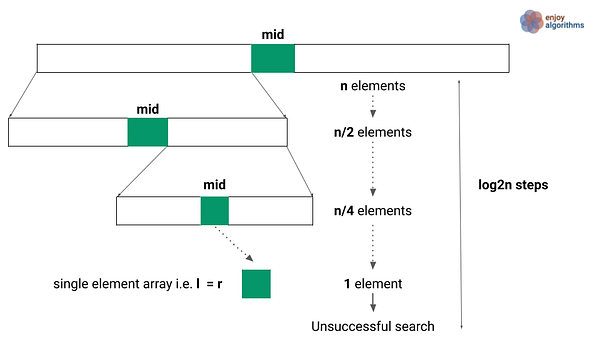=> n -> n/2 -> n/4 -> …… 1 -> unsuccessful search

Suppose we reach the base case after k steps.

=> n/2^k = 1 => n = 2^k => k = log2n

So, binary search time complexity = O(logn)

Another idea of analysis !

For the clear picture, let’s assume for the moment that the size of the array is a power of 2 (n = 2^k => k = log2n). Now each time when we compare the middle element, we cut the size of the subarrays to search by half.

• Before the first step of the algorithm, the subarray size is 2^k.
• After the first step, now subarray size would be 2^(k-1).
• After the second step, now subarray size would be 2^(k-2).
• Let’s assume after some k steps, we reach the single element subarray. So it will be 2^(k-k)= 1. Now we will reach the base case in the next step. All together we can have at most k + 1 step or log2n + 1 step.
• Within each step, we perform a constant amount of work: calculating the mid-point and a comparison operation. So, overall, when given the array size n we perform c (log2 n + 1) operations.
• Time complexity = O(logn)

Binary search analysis using master theorem

Let’s assume that T(n) is the worst-case time complexity of the binary search for n elements. When we have n>0, then we can break down the time complexities as follows —

• Divide part : The time complexity of the divide part is O(1) because this step calculates the middle index of the array, which takes constant time.
• Conquer part : We are recursively solving one sub-problems of size n/2. So the overall time complexity of the conquer part is T(n/2).
• Combine part : As mentioned above, this part is trivial. So time complexity of the combine part is O(1).

For calculating the T(n), we need to add the time complexities of the divide, conquer, and combine part => T(n) = O(1) + T(n/2) + O(1) = T(n/2) + c. Here is the recurrence relation of the worst-case time complexity:

• T(n) = c, if n = 1
• T(n) = T(n/2) + c, if n > 1

This recurrence relation is in the form T(n) = aT(n/b) + O(n^k) where a≥1 and b>1. We can think to apply the master theorem! There are the three cases of the solution via master theorem:

1. If f(n) = O(n^k) where k < logb(a) then T(n) = O(n*logb(a))
2. If f(n) = O(n^k) where k = logb(a) then T(n) = O(n^k * logn)
3. If f(n) = O(n^k) where k > logb(a) then T(n) = O(f(n))

If we compare the recurrence relation of binary search and master theorem

• T(n) = T(n/2) + c
• T(n) = aT(n/b) + O(n^k)

a = 1, b = 2 where both a≥1 and b>1

=> f(n) = c = cn^0 = O(n^0) => k = 0

=> Similarly, logb(a) = log 2(1) = 0 => Hence, logb(a) = k = 0

So binary search recurrence satisfy the 2nd case of the master theorem. Time complexity T(n) = O(n^k logn) = O(n^0 logn) = O(logn)

### Binary search algorithm space complexity

The space complexity of the binary search depends on the implementation. In the iterative approach, we use constant extra space so that the space complexity would be O(1). In the recursive method, space complexity depends on the size of the recursion call stack.

• Recursion call stack size depends on the total number of levels in the recursion tree or the height of the recursion tree.
• The height of the recursion tree is equal to logn + 1. From top to down till base case, at each level, we have only one recursive call!
• The space complexity of the recursive implementation = O(log n)

### An interesting story from history!

Here is a note by Jon Bentley about binary search from the book Programming Pearls:

I’ve assigned binary search in courses at Bell Labs and IBM. Professional programmers had a couple of hours to convert its description into a program in the language of their choice; high-level pseudocode was fine. At the end of the specified time, almost all the programmers reported that they had the correct code for the task. We would then take thirty minutes to examine their code, which the programmers did with test cases. In several classes and with over a hundred programmers, the results varied little: ninety percent of the programmers found bugs in their programs (and I wasn’t always convinced of the correctness of the code in which no bugs were found). I was amazed: given ample time, only about ten percent of professional programmers were able to get this small program right. But they aren’t the only ones to find this task difficult: in Section 6.2.1 of his Sorting and Searching history, Knuth points out that while the first binary search was published in 1946, the first published binary search without bugs did not appear until 1962.

### Critical ideas to think!

• Why did we not use the equation (left + right)/2 to calculate the mid index? Here is the reason: Practically, for the large value of the left and right index, the value of (left + right) may exceed the range of integers of the data type (even if left and right are within the range). This can result in an integer overflow for very large arrays. That’s why we have used the equation mid = left + (right-left)/2 to fix the integer overflow error. You can follow this wonderful reference for better understanding — https://ai.googleblog.com/2006/06/extra-extra-read-all-about-it-nearly.html
• Most of the programmers who incorrectly implemented binary search made an error in defining the base condition of recursive code or exit condition of iterative code. Think!
• For small array sizes, linear search is faster than binary search! Why?
• We can use the idea binary search idea to solve several coding problems where the array has some order property similar to the sorted array. For example, for a given value, How can we modify the binary search to find the count of smaller elements, the value of the next-smallest element, the value of the next-largest element, and all nearest neighbors?
• Even if there are duplicate elements in the array, a binary search may return an index whose value equals the target value. Still, it does not always return the first or last occurrence of the element. Can we modify the binary search to find the first or last occurrence of the element? Think!
• On sorted arrays, binary search will not work efficiently when insertion and deletion are frequently happening with searching. The insertion and deletion operations will take O(n) time in the case of a sorted array. Think!
• Exponential search is a variation of binary search for the unbounded array, i.e., an array for which the rightmost boundary is unknown. It can also work perfectly on the bounded array, but efficiency is only better than binary search if the target value lies near the start of the array. Think!
• In a binary search tree, data are arranged in sorted order of the tree structure, and each data can be searched using an idea similar to binary search. Insertion and deletion also work perfectly, requiring average O(logn) time, which is faster than insertion and deletion of sorted arrays.

### Binary search-based problems to practice

Enjoy learning, Enjoy coding, Enjoy algorithms!

Subscribe to get well-designed content on data structures and algorithms, machine learning, system design, oops, and mathematics. enjoy learning!

### We Welcome Doubts and Feedback!